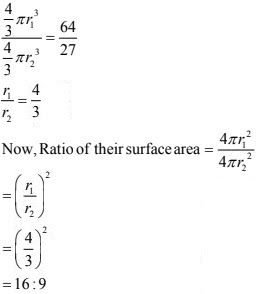# Chapter 12 Surface Areas and Volumes NCERT Exemplar Solutions Exercise 12.1 Class 10 Maths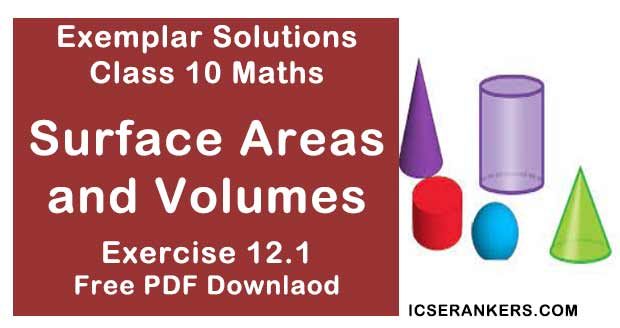Chapter Name NCERT Maths Exemplar Solutions for Chapter 12 Surface Areas and Volumes Exercise 12.1 Book Name NCERT Exemplar for Class 10 Maths Other Exercises Exercise 12.2Exercise 12.3Exercise 12.4 Related Study NCERT Solutions for Class 10 Maths

### Exercise 12.1 Solutions

Multiple Choice Questions:

Choose the correct answer from the given four options:

1. A cylindrical pencil sharpened at one edge is the combination of
(A) a cone and a cylinder
(B) frustum of a cone and a cylinder
(C) a hemisphere and a cylinder
(D) two cylinders

Solution

(A) a cone and a cylinder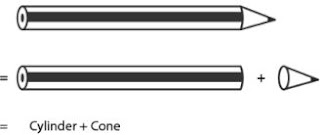The Nib of a sharpened pencil = conical shape
And,
The rest of the part of a sharpened pencil = cylindrical
Therefore, a pencil is a combination of cylinder and a cone.

2. A surahi is the combination of:
(A) a sphere and a cylinder
(B) a hemisphere and a cylinder
(C) two hemispheres
(D) a cylinder and a cone
Solution
(A) a sphere and a cylinder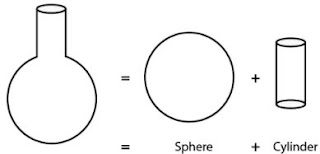The top part of surahi = cylindrical shape
Bottom part of surahi = spherical shape
Therefore, surahi is a combination of Sphere and a cylinder.

3. A plumbline (sahul) is the combination of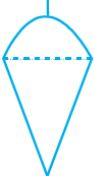(A) a cone and a cylinder
(B) a hemisphere and a cone
(C) frustum of a cone and a cylinder
(D) sphere and cylinder
Solution
(B) a hemisphere and a cone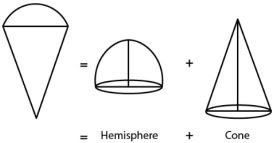The upper part of plumb line = hemispherical,
The bottom part of plumb line = conical
Therefore, it is a combination of hemisphere and cone.

4. The shape of a glass (tumbler) is usually in the form of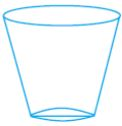(A) a cone
(B) frustum of a cone
(C) a cylinder
(D) a sphere
Solution
(B) frustum of a cone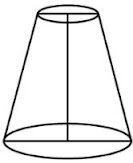The shape of glass is a frustum or specifically, an inverted frustum.

5. The shape of a gilli, in the gilli-danda game, is a combination of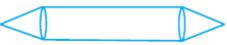(A) two cylinders
(B) a cone and a cylinder
(C) two cones and a cylinder
(D) two cylinders and a cone
Solution
(C) two cones and a cylinder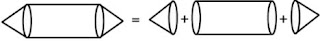The left and right part of a gilli = conical
The central part of a gilli = cylindrical
Therefore, it is a combination of a cylinder and two cones.

6. A shuttle cock used for playing badminton has the shape of the
combination of
(A) a cylinder and a sphere
(B) a cylinder and a hemisphere
(C) a sphere and a cone
(D) frustum of a cone and a hemisphere
Solution
(D) frustum of a cone and a hemisphere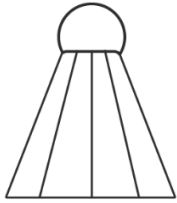The cork of a shuttle = hemispherical shapes
The upper part of a shuttle = shape of frustum of a cone.
Therefore, it is a combination of frustum of a cone and a hemisphere.

7. A cone is cut through a plane parallel to its base and then the cone that is formed on one side of that plane is removed. The new part that is left over on the other side of the plane is called
(A) a frustum of a cone
(B) cone
(C) cylinder
(D) sphere
Solution
(A) a frustum of a cone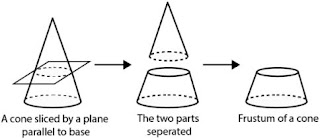When a cone is divided into two parts by  a plane through any point on its axis parallel to its base, the upper and lower parts obtained are cone and a frustum respectively.

8. A hollow cube of internal edge 22cm is filled with spherical marbles of diameter 0.5 cm and it is assumed that 1/8 space of the cube remains unfilled. Then the number of marbles that the cube can accommodate is
(A) 142296
(B) 142396
(C) 142496
(D) 142596
Solution
(A) 142296
According to the question,
Volume of cube =223=10648cm3
Volume of cube that remains unfilled =1/8×10648
=1331cm3
Volume occupied by spherical marbles =10648−1331
= 9317cm3
Radius of the spherical marble = 0.5/2
= 0.25cm
= 1/4cm
Volume of 1 spherical marble = (4/3) × (22/7) × (1/4)3
= 11/168 cm3
Numbers of spherical marbles, n = 9317 × (11/68)
= 142296

9. A metallic spherical shell of internal and external diameters 4 cm and 8 cm, respectively is melted and recast into the form a cone of base diameter 8cm. The height of the cone is
(A) 12cm
(B) 14cm
(C) 15cm
(D) 18cm
Solution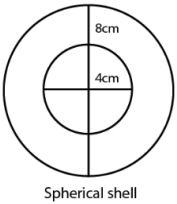Volume of spherical shell = Volume of cone recast by melting
For Spherical Shell,
Internal diameter,
d1 = 4 cm
r1 = 2 cm
External diameter,
d2 = 8 cm
r2 = 4 cm
Now,
As volume of spherical shell = [4π/3(r23 - r13)]
Where r1 and r2 are internal and external radii respectively.
Volume of given shell = [4π/3(43 - 23)]
= [(4π/3) × 56]
= (224/3)π
We know that,
Volume of cone = (224/3)π cm3
For cone,
Base diameter = 8 cm
Base radius, r = 4 cm
Let Height of cone = 'h'..
We know,
Volume of cone = (1/3)π42 h
224π/3 = 16πh/3
⇒ 16h = 224
⇒ h = 14 cm
So, Height of cone is 14 cm.

10. A solid piece of iron in the form of a cuboid of dimensions 49cm × 33cm × 24cm, is molded to form a solid sphere. The radius of the sphere is
(A) 21cm
(B) 23cm
(C) 25cm
(D) 19cm
Solution
AS we know,
Volume of cuboid = lbh
Where,
l = length,
h = height
For given cuboid,
Length,
l = 49 cm
b = 33 cm
Height,
h = 24 cm
Volume of cube  = 49 × 33 × 24 cm3
Now, Now
Let the radius of cube be r.
As volume of sphere  = (4/3)πr3 Where r = radius of sphere
Also,
Volume of cuboid = volume of sphere molded
49(33)(24) = (4/3)πr3
⇒ πr3 = 29106
⇒ r3 = 29106 × 22/7
⇒ r3 = 9261 = 21 cm
Hence, radius of sphere is 21 cm

11. A mason constructs a wall of dimensions 270cm× 300cm × 350cm with the bricks each of size 22.5cm × 11.25cm × 8.75cm and it is assumed that 1/8 space is covered by the mortar. Then the number of bricks used to construct the wall is
(A) 11100
(B) 11200
(C) 11000
(D) 11300
Solution
(B) 11200
Volume of wall = 270cm× 300cm × 350cm
=28350000 cm3
1/8 space is covered by the mortar,
Remaining space of wall = Volume of wall - volume of mortar
= 28350000 - 28350000 × 1/8
= 24806250
Now,
Volume of 1 brick = 22.5 cm  × 11.25 cm × 8.75 cm
= 2214.844 cm3
So,
Required no. of bricks,
= 24806250/2214.844
= 11200

12. Twelve solid spheres of the same size are made by melting a solid metallic cylinder of base diameter 2 cm and height 16 cm. The diameter of each sphere is
(A) 4 cm
(B) 3 cm
(C) 2 cm
(D) 6 cm
Solution
(c) Given,
Diameter of the cylinder = 2 cm
Height of the cylinder = 16 cm  [diameter = 2 × radius]
Volume of the cylinder = π × (1)2 × 16
= 16π cm3
According to question,
Volume of 12 solid spheres = Volume of cylinder
⇒ 12 × (4/3)πr3 = 16π
⇒ r = 1
Diameter of each sphere, d = 2r
= 2× 1 = 2 cm
Hence, the required diameter of each sphere is  2 cm.

13. The radii of the top and bottom of a bucket of slant height 45 cm are 28 cm and 7 cm, respectively. The curved surface area of the bucket is
(A) 4950 cm2
(B) 4951 cm2
(C) 4952 cm2
(D) 4953 cm2
Solution
(a) Given,
The radius of the top of the bucket,
R = 28 cm
The radius of the bottom of the bucket,
r = 7 cm
Slant height of the bucket,
l = 45 cm
Since, bucket is in the form of frustum of a cone.
Curved surface area of the bucket = = πl(R + r)
= π × 45 (28 + 7)
= 4750 cm2

14. A medicine-capsule is in the shape of a cylinder of diameter 0.5 cm with two hemispheres stuck to each of its ends. The length of entire capsule is 2 cm. The capacity of the capsule is
(A) 0.36 cm3
(B) 0.35 cm3
(C) 0.34 cm3
(D) 0.33 cm3
Solution
(A) 0.36 cm3
Given, diameter of cylinder = Diameter of hemisphere = 0.5 cm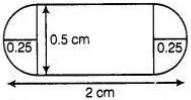Total length of capsule = 2 cm
So,
Length of cylindrical part of capsule  = 2 - (0.25 + 0.25) = 1.5 cm
So,
Capacity of capsule = Volume of cylindrical part + 2 × volume of hemisphere
= πr2h + (2/3)πr3
= 0.36 cm3

15. If two solid hemispheres of same base radius r are joined together along their bases, then curved surface area of this new solid is
(A) 4πr2
(B) 6πr2
(C) 3πr2
(D) 8πr2
Solution
(A) 4πr2
Because curved surface area of a hemisphere is 2πr2 and here, we join two solid hemispheres along their bases of radius r, from which we get a solid sphere.
Hence, the curved surface area of new solid = 2πr2 + 2πr2 = 4πr2

16. A right circular cylinder of radius r cm and height h cm (h>2r) just encloses a sphere of diameter
(A) r cm
(B) 2r cm
(C) h cm
(D) 2h cm
Solution
(B) 2r cm
Because the sphere encloses in the cylinder, therefore the diameter of sphere is equal to
diameter of cylinder which is 2r cm.

17. During conversion of a solid from one shape to another, the volume of the new shape will
(A) increase
(B) decrease
(C) remain unaltered
(D) be doubled
Solution
(C) remain unaltered
During conversion of a solid from one shape to another, the volume of the new shape will
remain unaltered.

18. The diameters of the two circular ends of the bucket are 44 cm and 24 cm. The height of the bucket is 35 cm. The capacity of the bucket is
(A) 32.7 litres
(B) 33.7 litres
(C) 34.7 litres
(D) 31.7 litres
Solution
(A) 32.7 litres
Given,
Diameter of one end of the bucket = 44cm
Diameter of other end of the bucket = 24cm
h = 35cm
Capacity of bucket = Volume of frustum of cone
= (1/3)πh(R2 + r2 + rR)
Putting value of R and r, we get,
Capacity of bucket = 32.71

19. In a right circular cone, the cross-section made by a plane parallel to the base is a
(A) circle
(B) frustum of a cone
(C) sphere
(D) hemisphere
Solution
(B) We know that, if a cone is cut by a plane parallel to the base of the cone, then the portion between the plane and base is called the frustum of the cone.

20. Volumes of two spheres are in the ratio 64:27. The ratio of their surface areas is
(A) 3 : 4
(B) 4 : 3
(C) 9 : 16
(D) 16 : 9
Solution
(D) 16 : 9
Let the radii of the two spheres are r1 and r2 respectively.
Therefore,
Ratio of volume of spheres :